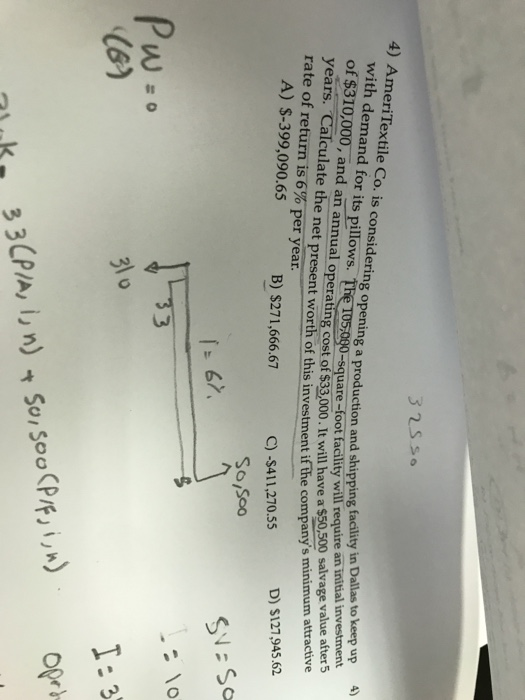# 4) AmeriTextile Co. is considering opening a production and shipping with demand for its pillows. T facility in Dal...4) AmeriTextile Co. is considering opening a production and shipping with demand for its pillows. T facility in Dallas to keep up uare-foot facility will require an initial investment and an annual operating cost of \$33,000. It will have a \$50,500 salvage va i lue after 5 Calculate the net present worth of this investment if the company's minimum years. rate of return is 6% per year. A) \$-399,090.65 D) S127,945.62 B) \$271,666.67 C) -\$411,270.55 So/Soo SVa Sa 3 T:3 /A, in)

Solution:

Let the cost of initial investment, IC = \$310,000

Annual operating cost, OC = \$33,000

Salvage value, SV = \$50,500 after N = 5 years, i = 6% per year

Net present worth, NPW = = - IC - OC*(P/A,i,N) + SV*(P/F,i,N)

NPW = - 310000 - 33000*(P/A, 0.06, 5) + 50500*(P/F, 0.06, 5)

Now, (P/A, 0.06, 5) = ((1+0.06)5-1)/(0.06*(1+0.06)5) = 0.338/0.08 = 4.212

(P/F, 0.06, 5) = (1+0.06)-5 = 0.747

So, NPW = - 310000 - 33000*4.212 + 50500*0.747

NPW = - 310000 - 138995 + 37725 = -411270 (approximately)

Thus, correct option is (C) -\$411,270.55

##### Add Answer of: 4) AmeriTextile Co. is considering opening a production and shipping with demand for its pillows. T facility in Dal...
More Homework Help Questions Additional questions in this topic.